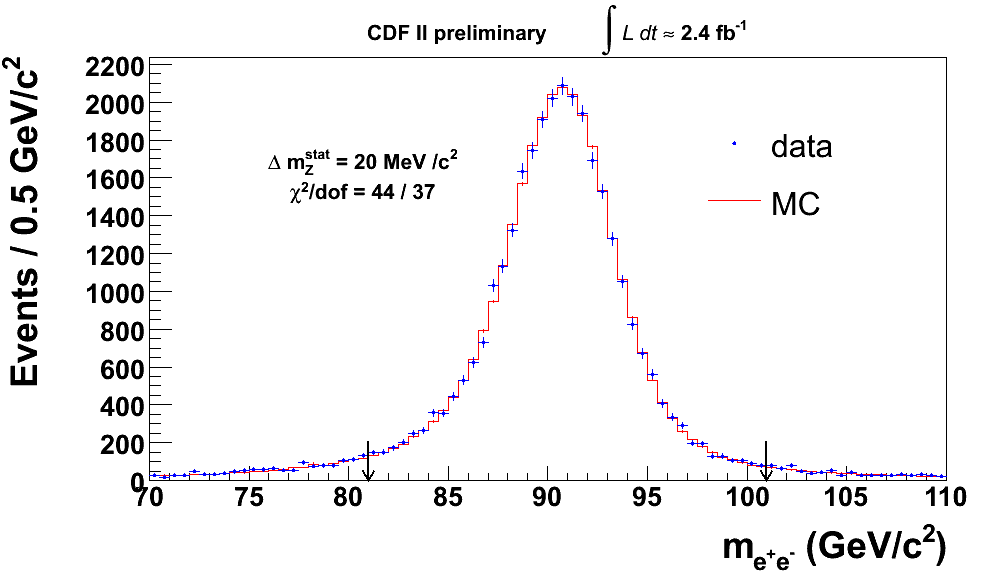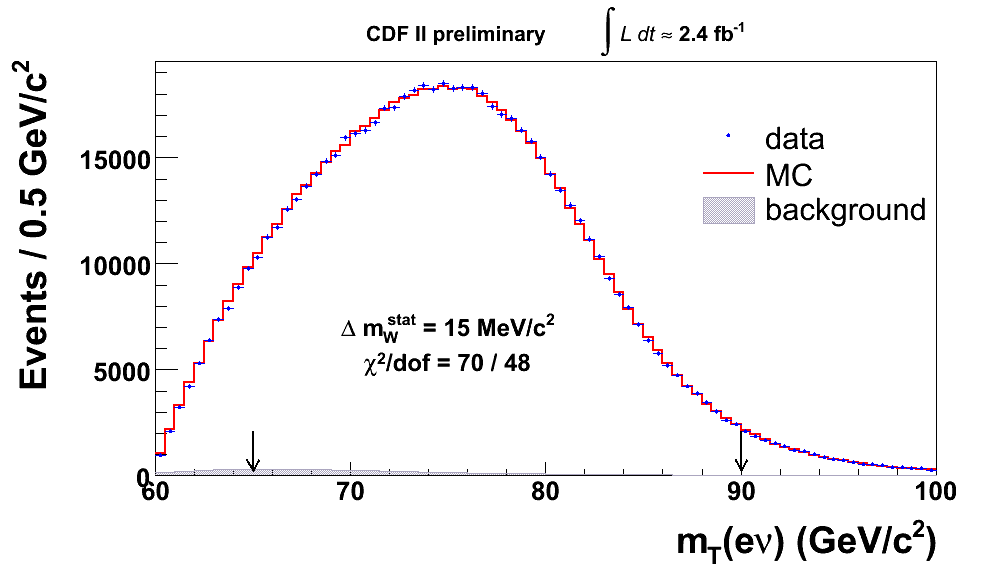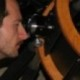## Toward a 2.3/fb W mass measurement August 21, 2008

Posted by dorigo in news, physics, science.
Tags: , ,

I looked with pleasure today at a few plots in the public web page of the CDF W mass measurement. They excellently describe the statistical power of the data collected so far by the experiment, and provide a clear hint that the next W mass measurement by CDF will make all previous determinations of this quantity grossly outdated, to say the least.

The W mass measurement is one of the most complicated analyses at a hadron collider. I would actually venture to say it is the most complicated one, and threaten anybody who wishes to challenge that judgement that I will use the argument “you object because you do not understand it well enough”, and proceed with showing why that is the case.

The reason why a measurement in HEP is more complicated than another one, in my opinion, is largely due to the potential of the data. When one has few events to study, statistics seriously limits the possible precision which can be obtained on any observable quantity derived from them, and a detailed study of systematics effects is useless. When one instead has to deal with huge statistics, the problem becomes that of understanding the systematic effects which affect the measurement, reducing them to a size comparable with the statistical uncertainty. If systematic uncertainties themselves can be studied with large samples of data, the level of understanding, and thus the level of detail of the studies, has to increase.

In the case of the W boson mass, the analysis was already extremely complex in Run I, when CDF had 100 inverse picobarns to study, and a few tens of thousands of good candidates of both the$W \to \mu \nu$ and the$W \to e \nu$ decays. I remember that in 1999 Andrew Gordon, a Harvard University graduate student who was doing the electron W mass measurement, took two years to try and figure out why the E/p distribution of electron tracks indicated one W mass value, and the$Z \to ee$ reconstructed mass pointed to another one which deviated by four standard deviations from the former. Gordon was one of the brightest students that ever walked the corridors of the CDF trailers, and yet the puzzle went unexplained. Or so I remember (anybody out there reading this, who know the solution?).

You might be wondering what the heck I am talking about. Well, let us see if I can explain it in a paragraph. Measuring the W mass from$W \to e \nu$ decays requires you to set the scale of the electron energy measurement. Setting the scale means, literally, converting a given signal read in the electromagnetic calorimeter -a phototube output- to a precise energy measurement in GeV. From the latter, the W mass is easy to extract. Well, easy… But let me focus on the scale now. There are two ways to determine precisely the scale of electron signals. One is to take a high-Et electron, measure its momentum in the tracker, and impose that the E/p distribution has the same shape expected from a tuned Monte Carlo (which must know everything about the amount of material traversed by the track, about internal QED radiation, about delta rays… You name it). By tweaking the energy measurement until an agreement of the shape with MC is reached, one determines the energy scale. The momentum, incidentally, is very well determined with other means, which do not interest us here.

I said one paragraph, ok let’s have two. The other method to set the scale of the e.m. calorimeter is to use the$Z \to ee$ decay: we know very well the mass of the Z boson, so a direct reconstruction of the Z peak from those events allow to tune the energy scale such that the Z mass distribution agrees with what is expected. Mind you, the peak does not come exactly at the Z mass value you find in the PDG (91.19 GeV, as measured by LEP): it comes out lower, because of parton distribution functions, internal QED radiation, and other effects. But with a good Monte Carlo simulation, those effects can be constrained, and the scale is determined well. How well? It depends on the statistics. There are fewer Z boson decays to dielectron pairs than there are electrons with which to determine the E/P distribution, so the Z mass tuning is intrinsically less powerful a method than the E/P.

Anyway, I was discussing the new results by CDF, which are based on 2.3 inverse femtobarns of proton-antiproton collisions. That is a huge number of W boson decays! The$p \bar p \to W \to e \nu$ process has a cross section of about three nanobarns, which means 3,000,000 femtobarns. You multiply by 2.3/fb and you get 7 million electron W events! This statistics is huge, and the accuracy which is required in understanding systematic effects is seriously challenging the CDF experts who are trying to produce a new world’s best W mass determination.

Let me remind you here, incidentally, that the W mass is now the most important input in the electroweak fits which attempt at constraining the mass of the Higgs boson in the Standard Model. See here for a short review of the current status of affairs.

Now, what I wanted to discuss in this post -which has become way longer than I wished- was just a couple of plots, which show the behavior of high-energy electrons as they cross the CDF detector.The plot above shows, for 2.3/fb of data, the Z boson mass reconstructed with electron energy deposits in the calorimeter. The shape is not exactly easy to describe analytically (it is a Breit-Wigner resonance shape convoluted with a gaussian resolution smearing, convoluted with PDF of quarks and antiquarks in the proton, and smeared with other small effects), but to a quick glance it looks roughly a gaussian peak.

Now look at the plot below, which shows, for the same data, the Z mass reconstructed by using the track momentum measured in the tracker, for those same electrons. What is going on ?Before you start thinking that the tracker measures momenta less well than the calorimeter measures energy, let me get this straight: the momentum in the tracker is measured with better accuracy -and in fact, that is the reason why setting the energy scale with the E/P distribution, using the momentum as a reference, is a valid method. The reason for the odd-shaped curve is bremsstrahlung: high-energy electrons traveling in the material of the CDF tracker emit soft photons, which can be collected and measured in the calorimeter, but are instead lost in the track measurement. For that reason, the Z mass distribution above has a long “radiative” tail to lower masses.

Now, look at the E/p distribution itself (see below). In absence of QED radiation, this would be a distribution peaking at one, with no tails at high values. Instead it does show a long tail, which is exactly the reason for the low-mass tail in the Z mass distribution above. Incidentally, look at the agreement with the simulation in the plot: amazing match! The residual systematic uncertainty coming from setting the energy scale with the E/P distribution, on the future W mass measurement, amounts to barely 5 MeV!And finally, a plot showing the W transverse mass distribution, which is obtained by using the electron energy as well as the missing transverse energy due to the escaping neutrino.The statistics is tremendous, and so is the level of agreement with the data. The statistical error on the W mass which can be fit with these events amounts to 15 MeV – which explains the amount of care which is needed to study all systematics. Incidentally, the$W \to \mu \nu$ final state provides a similar statistical accuracy, and once the two measurements are combined, the total uncertainty in the W mass measurement will be in the 20 MeV ballpark.1. fliptomato - August 22, 2008

Hi Tommaso — this is a really helpful post! A few questions:

1. If I understand correctly, then you use$Z\rightarrow ee$ to calibrate the electromagnetic calorimeter? Then this calibration is used to identify the energy of the electron in$W\rightarrow e\nu$?

What about hadronic recoil? E.g.$q\overline q \rightarrow g,Z \rightarrow g,e\nu$? This process is not so suppressed, especially when the gluon is close to collinear with the beam and one gets a factor of the strong coupling and a Sudakov logarithm. One would thus have a$W$ with nonzero transverse momentum and some hadronic jet. Isn’t it then important to somehow model the jet so that an accurate determination of the$W$ mass/width can be done?

I naively thought (reading one of the CDF papers, arXiv:0707.0085) that measuring the$Z$ branching ratio had to do with some kind of modeling of the hadronic transverse momentum?

[My understanding is that plotting$d\sigma/dm_T$ instead of$d\sigma/dp_T$ helps in this case, but it’s not obvious to me how this works.]

2. A very naive question: when you say that you look at the E/p distribution, which E and p are you using? Is this the electron E/p? Does this mean we’re comparing the energy deposited in the calorimeter compared to the momentum extracted from tracking?

When you explained the tail on the E/p distribution, you said this was because of bremsstrahlung of high-energy electrons. So the sot photons count towards the energy in the calorimeter, but not the curving in the tracking used to fit the momentum. Since the tracking system is closer to the beam pipe than the calorimeter, are you saying that the bremsstrahlung occurs nearly instantaneously so that the electron radiates away photons before drifting through the tracking chamber?

Also, how do you know what the momentum$p$ is in the expression$E/p$? Presumably energy of an electron can be measured in the EM calorimeter, but we can’t determine$p$ since we don’t know the longitudinal component of momentum? (Or do we just use the information from the pseudorapidity of the deposited energy in the calorimeter?)

3. In your last plot of the distribution with respect to transverse mass, can you explain why the shape takes this form? My understanding is that there’s a Jacobian peak that is smoothed out by various effects. Why is it that the `smoothing’ out causes the lower values of$m_T$ to be enhanced relative to the Jacobian peak?

Thanks very much!
Flip2. Markk - August 22, 2008

Hi,
I am always awed by those fitted curves you present where the prediction is so close to real measures. A generic question about what you mean when you say Monte Carlo here. What exactly are you simulating?

Do you have a physical model of the interaction region and the detectors and are running events like actual collisions, where you get outputs that look like collision pictures? Or do you have an interaction model for a particular detector and run off of a distribution for that? (e.g. a distribution of voltage values or temperature readings) Or both? I could see where putting several of the second kinds of run together might give something different than the first.3. dorigo - August 22, 2008

Hi Flip,

Using the Z to calibrate the calorimeter response to 40 GeV electrons is a good way but not the preferred one. The E/P method is more precise.

The recoil modeling is very important in the W mass measurement. In fact, it is one of the most important parts of the study. The recoil is modeled on Z->ee and Z->mm decays, and then the Monte Carlo is used to extrapolate to the W.

The Z->ll BR can be measured only in the ratio$\frac {\sigma_W B(e \nu)}{\sigma_Z B(ee)}$ (it is about 10). Through it one can get a direct measurement of the W width, by the way.

Using the transverse mass is better than using the lepton Pt because of the recoil: the transverse mass is less sensitive on the recoil modeling – although the neutrino missing Et is then to be measured accurately.

Yes, the E/p relate to the electron measurement.

No, the bremsstrahlung occurs whenever the electron passes close to heavy-Z atoms. This occurs throughout its path. The soft photons get collected in a cluster of calorimeter energy, and are measured together with the electron shower.

The tracker measures the five parameters of a track helix. There is a 1.4 Tesla axial magnetic field, and there are stereo layers in the tracker that allow z-coordinate measurements. Track momenta are tuned precisely with J/psi mesons, Y mesons, Z bosons, to a very good accuracy (see the recent mass measurement of the X meson, I discussed it a week ago here).

When you reconstruct a transverse mass, you lose the z component of momenta. So the Mt is strictly smaller than the true M. That explains the skewed jacobian peak. The precise distribution depends on how central W production is, and that depends on PDF, on detector acceptance, etcetera…

Hope that helps.
Cheers,
T.4. dorigo - August 22, 2008

Hi Markk,

no, the Monte Carlo is really a precise simulation if the physics of the collision – the interaction, the emitted particles, the hadronization, the decays in flight. Then there is a simulation of the interaction of these bodies with the detector material, and simulated readouts of the detector components. Some parts are parametrized, but most is simulated to the best of our knowledge. A Monte Carlo event looks exactly like a real one after reconstruction, except for the presence of an additional bank containing the particle level information.

Cheers,
T.5. Alejandro Rivero - August 27, 2008

The 2004 relativistic prediction/estimate from Hans de Vries leaves the ballpark (from pdf 2008 mass of Z) in the fork 80.3763..80.3726 GeV. Current pdg value, 80.398 pm 0.025, still intersects with the estimate, with some stress if you wish. As in the case of the top mass, it is not really important if the new value discrepates or not; de Vries theory does not account for corrections of the order of pi/alpha etc. But it will be interesting/amusing to see how far it comes.6. dorigo - August 27, 2008

Hi Alejandro,

sorry for the ignorance, but do you have a quick reference to HDV’s work on W mass predictions ?

Cheers,
T.7. Alejandro Rivero - August 27, 2008

Dorigo, do not worry, it is just one of out collection of numerical guessing in http://www.physicsforums.com. In this case, Hans did the relativistic De Broglie approximation for a circular orbit in two cases, for total angular momentum L=1/2 and L=1 (translated to quantum sqrt(L(L+1)) and then he quotients the orbital speed, or frequencies if you wish, so that he gets and adimensional number, independent of the orbital radius. Damn me if I know why Hans did it, it is just very good in the work of getting a formula for a result. But yep, the quotient is as good a Weinberg cosine as you can get.8. dorigo - September 1, 2008

Oh, I see. I even think I ran into the explanation once! Yes I did. I’m just growing old and retarded.

Cheers,
T.9. fliptomato - September 2, 2008

Hi again Tommaso — is there a paper associated with the new plots, or should we just refer to the 2007 paper?

-F10. dorigo - September 2, 2008

Yes Flip, no completed analysis yet, no paper. Once there is an updated Mw measurement, there also will be a new paper…
T.

Sorry comments are closed for this entry